##### 3  Extended approaches
[German version]

###### 3.2 Deformation and force development of securing devices

Breaking the direction of action of securing devices down into Cartesian components has already been presented in section 2. In geometric terms, each securing device has the components X, Y and Z assigned to it, with the Pythagorean relation to its length L:Cargo movement or deformation is expressed by specific, small changes DX, DY and DZ in these components. Change in length DL is then precisely calculated by: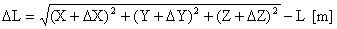If the individual changes are small relative to the total length L, the change in length DL may in most cases sufficiently accurately also be determined by an approximation equation as follows: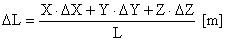This approximation equation should, however, not be used if for example component Y is close to zero and a cargo shift involving a DY is to be investigated. This case in particular applies to steep tie-down lashings.

If force development by securing devices as a result of cargo movement or deformation is to be determined, it is advisable to assign a "personal" factor to each securing device which makes it possible to calculate the force change DF directly from the change in length DL. This factor is the spring constant D conventionally used in industrial mechanics. The relation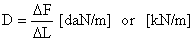applies.

The spring constant includes the cross-section, modulus of elasticity and length of the securing device as influencing variables in accordance with Hooke’s law: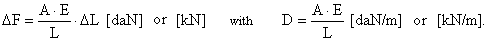DF = force change in the securing device [daN] or [kN]

A = cross-section of securing device [cm2]

E = modulus of elasticity [daN/cm2] or [kN/cm2]

L = loaded length of securing device [m]

DL = change in length of securing device [m]

The spring constant is not generally stated in data provided by manufacturers of securing equipment. There are several ways of determining the spring constant depending on the information which is available about the securing material.

Manufacturers of ropes, chains and belts often state that the elongation of the material is P % when the material is loaded with a specific force F (LC is usually stated here). The force F stated corresponds to the force change DF starting from zero load and assuming approximately linear load/elongation behavior, which is generally the case in the limited load range between pretension F0 and admissible load LC. The spring constant is thus: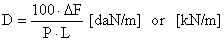If the length of the lashings is initially unknown, a normalized spring constant DN for the unit length of 1 m may be used for the lashing material, the following applying: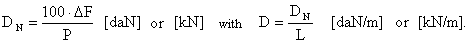The spring constants of pressure transferring element, e.g. squared lumber, may be estimated from the three variables cross-section A, modulus of elasticity E and length L: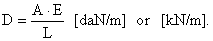The spring constant of end walls and stanchions on a truck loading area may be estimated by regarding them as beams clamped on one end. The spring constant is then calculated on the basis of the bending equation for cantilevers: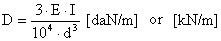E = modulus of elasticity [daN/cm2] or [kN/cm2]

I = geometrical moment of inertia in clamping point [cm4]

d = lever length of clamped beam [m]

In practice, however, this solution will provide excessively large values for D, since the wall or stanchion is not clamped absolutely rigidly, the load-bearing substructure instead likewise deforming. It is therefore advisable to establish a correction factor by making representative measurements or to determine the entire spring constant experimentally. The same also applies to sidewalls of truck loading areas which are differently designed and often receive some support over their length from the roof structure. A simple formula can no longer be stated in this case.

The following formula applies to devices arranged in parallel: D = D1 + D2 + … + Dn

The following formula applies to devices arranged in series: 1/D = 1/D1 + 1/D2 + … + 1/Dn